# ISEE Middle Level Math : Fractions

## Example Questions

1 2 34 35 36 37 38 39 40 42 Next →

### Example Question #552 : Numbers And Operations

Divide the following: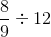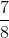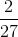Explanation:

To divide fractions, we will take the first fraction and multiply by the reciprocal of the second fraction.  So, we get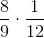We will simplify before we multiply to make things easier.  The 8 and the 12 can both be divided by 4.  We get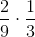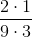### Example Question #561 : Numbers And Operations

Divide the following: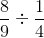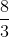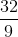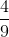Explanation:

To divide, we will multiply the first fraction by the reciprocal of the second fraction.  To find the reciprocal, the numerator becomes the denominator, and the denominator becomes the numerator (in other words, we will flip the fraction).

We get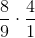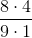### Example Question #412 : Fractions

Divide the following: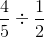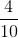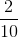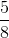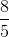Explanation:

To divide fractions, we will take the first fraction and multiply it by the reciprocal of the second fraction.

To find the reciprocal, the numerator will become the denominator, and the denominator will become the numerator.  In other words, we will flip the fraction.

So, we get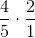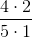### Example Question #413 : Fractions

Divide the following: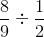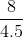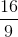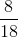Explanation:

To divide fractions, we will take the first fraction and multiply it by the reciprocal of the second fraction.  To find the reciprocal, the numerator will become the denominator, and the denominator will become the numerator.  In other words, we will flip the fraction.  So, we get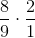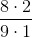### Example Question #414 : Fractions

Divide the following: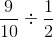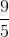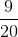Explanation:

To divide fractions, we will take the first fraction and multiply it by the reciprocal of the second fraction.  To find the reciprocal of a fraction, the numerator will become the denominator, and the denominator will become the numerator.  In other words, we will flip the fraction.

So, we get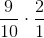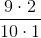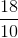### Example Question #411 : Fractions

Divide the following: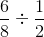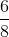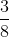Explanation:

To divide fractions, we will take the first fraction and multiply it by the reciprocal of the second fraction. To find the reciprocal of a fraction, the numerator will become the denominator, and the denominator will become the numerator. In other words, we will flip the fraction.

So, we get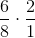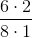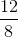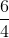1 2 34 35 36 37 38 39 40 42 Next →

### All ISEE Middle Level Math Resources